## Solving Equations With Integers Using Properties of Equality

### Learning Outcomes

• Solve equations using the addition and subtraction properties of equality
• Model the division property of equality
• Solve equations using the multiplication and division properties of equality

## Solve Equations with Integers Using the Addition and Subtraction Properties of Equality

In Solve Equations with the Subtraction and Addition Properties of Equality, we solved equations similar to the two shown here using the Subtraction and Addition Properties of Equality. Now we can use them again with integers.

$x+4=12$                                  $y-5=9$

$x+4\color{red}{-4}=12\color{red}{-4}$                    $y-5\color{red}{+5}=9\color{red}{+5}$

$x=8$                                            $y=14$

When you add or subtract the same quantity from both sides of an equation, you still have equality.

### Properties of Equalities

Subtraction Property of Equality Addition Property of Equality
$\text{For any numbers }a,b,c$,

$\text{if }a=b\text{ then }a-c=b-c$.

$\text{For any numbers }a,b,c$,

$\text{if }a=b\text{ then }a+c=b+c$.

### example

Solve: $y+9=5$

Solution

 $y+9=5$ Subtract $9$ from each side to undo the addition. $y+9\color{red}{-9}=5\color{red}{-9}$ Simplify. $y=-4$

Check the result by substituting $-4$ into the original equation.

 $y+9=5$ Substitute $−4$ for y $-4+9\stackrel{?}{=}5$ $5=5\quad\checkmark$

Since $y=-4$ makes $y+9=5$ a true statement, we found the solution to this equation.

### example

Solve: $a - 6=-8$

### try it

In the following video we show more examples of how to solve linear equations involving integers using the addition and subtraction properties of equality.

## Model the Division Property of Equality

All of the equations we have solved so far have been of the form $x+a=b$ or $x-a=b$. We were able to isolate the variable by adding or subtracting the constant term. Now we’ll see how to solve equations that involve division.

We will model an equation with envelopes and counters.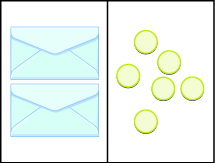Here, there are two identical envelopes that contain the same number of counters. Remember, the left side of the workspace must equal the right side, but the counters on the left side are “hidden” in the envelopes. So how many counters are in each envelope?

To determine the number, separate the counters on the right side into $2$ groups of the same size. So $6$ counters divided into $2$ groups means there must be $3$ counters in each group (since $6\div2=3$).

What equation models the situation shown in the figure below? There are two envelopes, and each contains $x$ counters. Together, the two envelopes must contain a total of $6$ counters. So the equation that models the situation is $2x=6$.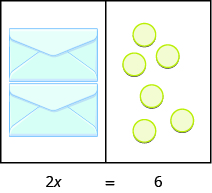We can divide both sides of the equation by $2$ as we did with the envelopes and counters.

$\Large{\frac{2x}{\color{red}{2}}=\frac{6}{\color{red}{2}}}$

$x=3$

We found that each envelope contains $\text{3 counters.}$ Does this check? We know $2\cdot 3=6$, so it works. Three counters in each of two envelopes does equal six.

Another example is shown below.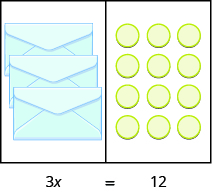Now we have $3$ identical envelopes and $\text{12 counters.}$ How many counters are in each envelope? We have to separate the $\text{12 counters}$ into $\text{3 groups.}$ Since $12\div 3=4$, there must be $\text{4 counters}$ in each envelope.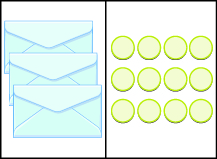The equation that models the situation is $3x=12$. We can divide both sides of the equation by $3$.

$\Large{\frac{3x}{\color{red}{3}}=\frac{12}{\color{red}{3}}}$

$x=4$

Does this check? It does because $3\cdot 4=12$.

### example

Write an equation modeled by the envelopes and counters, and then solve it.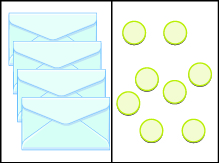Solution
There are $\text{4 envelopes,}$ or $4$ unknown values, on the left that match the $\text{8 counters}$ on the right. Let’s call the unknown quantity in the envelopes $x$.

 Write the equation. $4x=8$ Divide both sides by $4$. $\Large{\frac{4x}{\color{red}{4}}=\frac{8}{\color{red}{4}}}$ Simplify. $x=2$

There are $\text{2 counters}$ in each envelope.

### try it

Write the equation modeled by the envelopes and counters. Then solve it.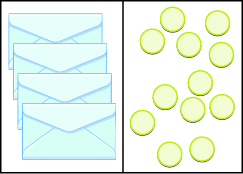$4x=12$; $x=3$

Write the equation modeled by the envelopes and counters. Then solve it.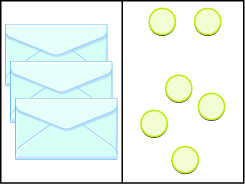$3x=6$; $x=2$

## Solve Equations Using the Division Property of Equality

The previous examples lead to the Division Property of Equality. When you divide both sides of an equation by any nonzero number, you still have equality.

### Division Property of Equality

$\begin{array}{ccc}\text{For any numbers}& a,b,c,\text{and}& c\ne 0,\\ \hfill \text{If}& a=b\text{ then}& \large{\frac{a}{c}=\frac{b}{c}}.\end{array}$

### example

$\text{Solve: }7x=-49$.

### example

Solve: $-3y=63$.

### try it

Watch the following video to see more examples of how to use the division and multiplication properties to solve equations with integers.